Courses

# Test: Signals And Systems & Microprocessors - 2

## 20 Questions MCQ Test GATE ECE (Electronics) 2022 Mock Test Series | Test: Signals And Systems & Microprocessors - 2

Description
This mock test of Test: Signals And Systems & Microprocessors - 2 for Electronics and Communication Engineering (ECE) helps you for every Electronics and Communication Engineering (ECE) entrance exam. This contains 20 Multiple Choice Questions for Electronics and Communication Engineering (ECE) Test: Signals And Systems & Microprocessors - 2 (mcq) to study with solutions a complete question bank. The solved questions answers in this Test: Signals And Systems & Microprocessors - 2 quiz give you a good mix of easy questions and tough questions. Electronics and Communication Engineering (ECE) students definitely take this Test: Signals And Systems & Microprocessors - 2 exercise for a better result in the exam. You can find other Test: Signals And Systems & Microprocessors - 2 extra questions, long questions & short questions for Electronics and Communication Engineering (ECE) on EduRev as well by searching above.
*Answer can only contain numeric values
QUESTION: 1

### A sequence x(n) with the z-transform X(z) = z3 + 2z2 - 3z + z-1 - 4z-3 is applied as an input to a linear, time-invariant system with the impulse response h(n) = 28(n - 2) where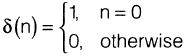The output at n = 2 is__________________________________

Solution:

The output y(n) can be drived as,
y(z) = I-1(z) X(z)

1.--1(z) = 2z-2

Y(z) = 2z-2 (z3 + 2z2 - 3z + z-1 - 4z-3) Y(z) 2(z + 2 - 3z-1 +

Taking inverse z-transform of Y(z) y(n) 2[6(n + 1) + 28(n) - 36(n - 1) +

(n - 3) -46(p - 511

At n = 2, y(2) = 0

QUESTION: 2

###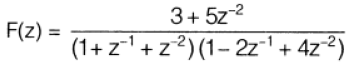then causal sequence f(n) is

Solution: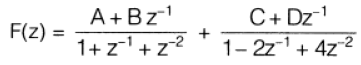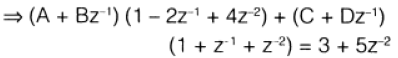On equating both sides we get. A 4- C = 3.

4A- 2B + C D = 5,

48 + D = 0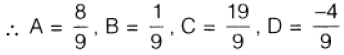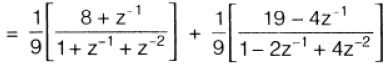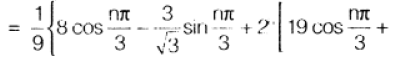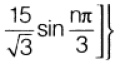QUESTION: 3

###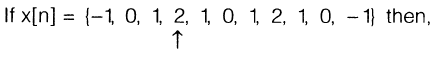Q. The value of X(eiπ) = ?

Solution:

Since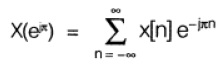∵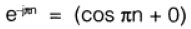= -1, when n = odd = +1, when rt = even

So,

X(e) = (2 +0+  2 + 0 +

[1 - 1 - 1 - 1 - 1 + 1]

4- 2 = 2

QUESTION: 4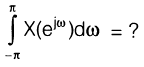Solution: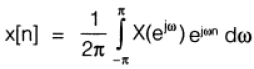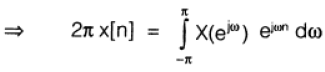at n 0, we have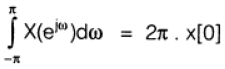= 2π X 2 = 4 π

QUESTION: 5

The Laplace transform of the waveform shown in the figure below is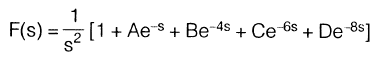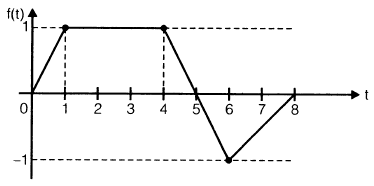Then what is the value of 'C'?

Solution:

From the waveform shown we have, f(t) t u(t) - (t - 1) u(t - 1) -

(t - 4) u(t - 4) + 1 .5(t - 6) u(t - 6)

- 1,5(t - 8) LAO - 8)

∴ F(s) = LT of f(t)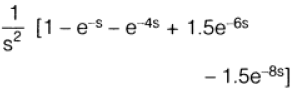Comparing this with the given Laplace transform and we get,

C = +1.5

QUESTION: 6

Fourier transform of the signal shown in figure below is,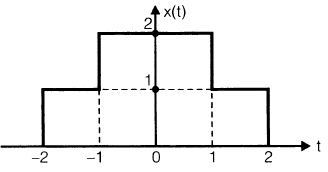Solution: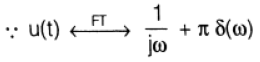Also, from the given plot we have

x(t) = u(t 2) + u(t + 1) - u(t - 1) - u(t - 2)

••• (I)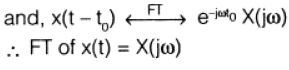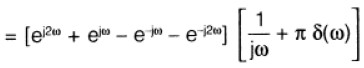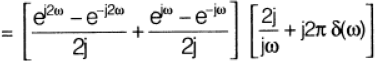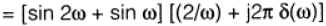QUESTION: 7

A DTS described by H(z) =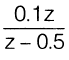when

excited by x[n] = cos(0.2 n + 10°) with discrete frequency Ω = 0.2.

Q. Find H(e) at Ω = 0.2 and is equals to

Solution:

For the given input x[n] cos 2n

response, y[n]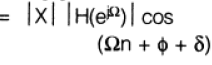Now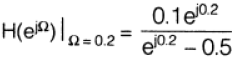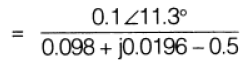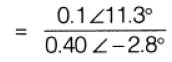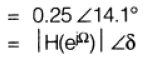QUESTION: 8

A DTS described by H(z) =when

excited by x[n] = cos(0.2 n + 10°) with discrete frequency Ω = 0.2.

Q. The steady state response y[n] is given by

Solution:

y[n] = 0.25 cos[0.2 n 10° + 14,1°) [n] = 0.25 cos[0.2 n +24.1°1

QUESTION: 9

Fourier analysis of waveform shown in figure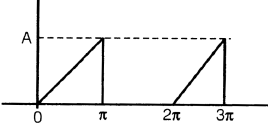Q. The waveform is

Solution:

wave form is neither odd nor even.

QUESTION: 10

Fourier analysis of waveform shown in figureQ.  In the waveform shown

1.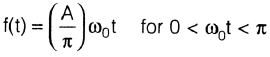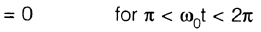2.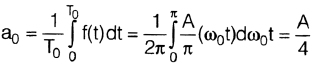3.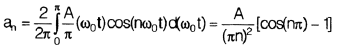4.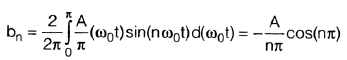5.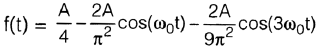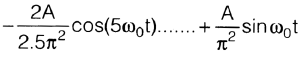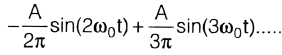Solution:
QUESTION: 11

Causal sequence f(n) if F(z) =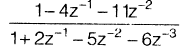, is given by

Solution: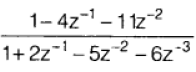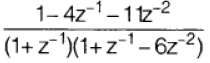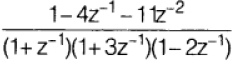........................(i)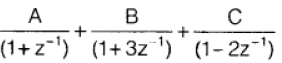........................(ii)

From 0) and 0) we get

A 1, B = 1, C = -1

Also we know, f(n) = Inverse z-transform of F(z)

f(n) = [(-1)n (-3)n - (2)n ] u (n)

QUESTION: 12

A signal x1(t) is having Fourier transform

Xi(co). Another signal x2(t) having Fourier transform X2(w) is related to Xi(co) by X2(w) = [1 +sg n(w)]Xi (co)

Value of x2(t) in terms of x1(t) will be

Solution: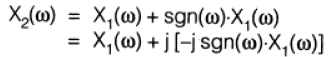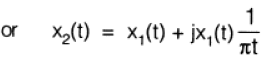QUESTION: 13

After some arithmetic operation flag register of 8085 microprocessor becomes BBH. The contents of the accumulator after the operation may be

Solution:

Flag registers in 808511P is as,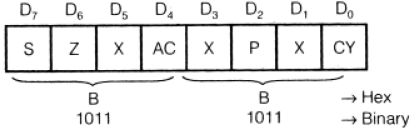Since, sign bit of 138.1-1. is 1 so, number is negative from the given choice only (c) or (d) gives the sign bit T.

Here, parity bit = 0 means total number of 1 's present is odd

So, only options (d) contains odd nu -r. her of 1's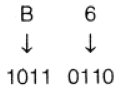Here five l's are present.

QUESTION: 14

The contents of register HL pair is 24FF H. Then the contents of register pair if, 1NR H and INR L operations are performed is

Solution:

∴  [H] <— 24
and [L] <— FF

INH H means increment the content of 'H' by 01.

∴          [H] <—  25

and       [L] <— FE

⇒ Content of H-L pair = 25FF H

L means increment the content of 'L'by 01.

∴    [H] <—  24

and    [L]   <— [FF] + 

i.e.  [L] <— 00

⇒ Content of IH-L pair = 2400 H

QUESTION: 15

If the HLT instruction of a 8085 microprocessor is executed then,

Solution:

HLT  →  is a 1-byte instruction.

→ processor stops executing and enters wait state

no register contents are affected.

the address bus and data bus are placed in high impedance state

QUESTION: 16

Line :                     MVI A,               B5H

MVI B,              OEH

XRI                   69H

ANI                    9BH

CPI                    9FH

STA                   3010 H

HLT

After execution of STA 3010H the status of Cy and Z flag will be

Solution:

Line    Cy        Z

1

2           0         0

3           0         0

4           0         0

0          0

6           1         0

7         1

QUESTION: 17

Three memory chips are of size 1 kB, 2 kB and 4 kB. Their address bus is 10 bits. The data bus sizes of chips are respectively

Solution:

∴ Memory size

= Add, bus size x Data bus size.

=(2)add bus line x.(Data bus size)

So, for 1 kB Chip.

Data bus size =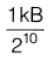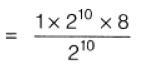For 2 kB chip,

Data bus size =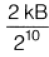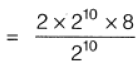16 bits

For 4 kB chip, Elata bus size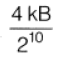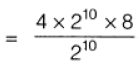= 32 bits

QUESTION: 18

An 8085 assembly language program is given below:

MV1C,           03H

LXI H,             2000 H

MOV             A, M

DCR

LOOP 1 : INX H

MOV B, M CMP B

JNC LOOP 2 MOV A, B

LOOP 2 : DCR C

JNZ LOOP 1 STA 2100 H HLT

Contents of memory location 2000: 18 H

2001 : 10H

2002 : 2BH

Q At the end of the program what will be the condition of carry and zero flags?

Solution:
QUESTION: 19

An 8085 assembly language program is given below:

MV1C,           03H

LXI H,             2000 H

MOV             A, M

DCR

LOOP 1 : INX H

MOV B, M CMP B

JNC LOOP 2 MOV A, B

LOOP 2 : DCR C

JNZ LOOP 1 STA 2100 H HLT

Contents of memory location 2000: 18 H

2001 : 10H

2002 : 2BH

What does the program do?

Solution:

Program is searching the value higher than the content of accumulator.

QUESTION: 20

Consider the given instructions of program for the delay register.

MVI           C, FFH

LOOP: DCR              C

JNZ           LOOP

Assume clock frequency of the system is 2 MHz.

Q.  The total T-states for this above programme is given by

Solution:

MVI C, FFH  →  7 T-states

LOOP: DCR C  → 5 T-states

JNZ LOOP → 10 T-states